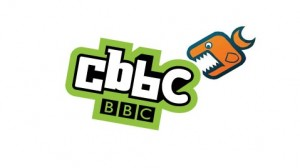# How to get a GCSE C grade by just using MyMaths and BBC Bite SizeBelow are a list of Key C grade topics that have links to lessons and revision pages on MyMaths and BBC Bite Size.

Obviously you will need a MyMaths login to access their pages but the BBC resources are free.

This extensive list and the corresponding pages will compliment any revision plan and should be used in conjunction with practice exam papers.If you would like some help with creating a revision time table click here for an article which has been shared several hundreds of times on twitter!

 TOPIC Grade Links Factors and Primes C/D HCF, prime factors, LCM,  BBC BITESIZE NUMBER Indices A-D Indices 1,  Harder indices (fractional and negative) Fractions, Decimals, Percentages C/D +-decimals, ordering decimals, x decimals by 10, 100, 1000, x decimals by whole numbers, x decimals together, divide decimals by whole numbers, equivalent fractions, fractions to decimals, +-fractions, x or divide fractions by a whole number, x fractions together, dividing fractions, Mixed numbers, Comparing fractions, % of amounts, fractions to decimals to percentages,  change as a % % change, C/D % change 1, % change 2 Rounding and estimation C/D significant figures, basic estimation, harder estimation, Ratio and Proportion C/D Dividing in a ratio, proportion Standard Form A/B standard form (large numbers), standard form (small numbers), standard form on calculators Algebraic expressions A-C Simplifying 1, simplifiying 2, solving equations, BBC BITESIZE ALGEBRA Sequences C nth terms Expanding brackets B-D single brackets, double brackets Factorising A-D factorising into 1 bracket, factorising into double brackets, harder double brackets Linear equations C/D y=mx+c, finding the equation of a line Straight-line graphs A-D co-ordinates, drawing graphs 3-D co-ordinates B/C 3-D co-ordinates Real-life graphs B-D real life graphs, distance-time graphs Formulae A-D substituting into formulae, rearranging formulae Inequalities A-C inequalities Quadratic graphs A-C plotting graphs Trial and improvement C trial and improvement Direct Proportion A-C direct and inverse proportion Angle properties C/D angles in parallel lines, angle proofs, BBC BITESIZE SHAPE, SPACE AND MEASURES Angles in polygons C/D interior and exterior angles, angles in a polygon Plans and Elevations C/D plans and elevations Perimeter and Area C/D area of a parallelogram, area of a triangle, area of a trapezium (formula given in exam!) Circles and cylinders C/D circumference, area, volume of cylinders Volumes of 3-D shapes A-D volume of prisms Pythagoras C Pythagoras Surface Area A-C nets and surface area Converting Units C/D converting units Units of area and volume B-D lengths v areas v volumes in formulae Speed, Density C/D speed, density Bearings C/D bearings Scale drawings C/D scale drawing, map scales Constructions C/D constructing triangles, perpendicular bisectors, angle bisectors etc Loci C loci Translations, rotations and reflections C/D rotations, reflections, translations, transformations Enlargements A-D enlargements Trigonometry B missing angles, missing sides Circle Theorems A circle theorems Collecting Data C/D Types of data, questionnaires, BBC BITESIZE HANDLING DATA Two-way tables C/D two-way tables Stratified Sampling A/B Sampling Mean, median and mode C/D comparing data using averages Frequency table averages C/D Grouping data, averages, mean from frequency tables Histograms A histograms Cumulative Frequency B c.f. 1, c.f. 2 Box plots B box plots Scatter graphs D scatter graphs, line of best fit Probability C/D listing outcomes, relative frequency, general probability revision, mutually exclusive events Tree diagrams A tree diagrams